JEE  >  WBJEE Physics Test - 2

# WBJEE Physics Test - 2

Test Description

## 40 Questions MCQ Test WBJEE Sample Papers, Section Wise & Full Mock Tests | WBJEE Physics Test - 2

WBJEE Physics Test - 2 for JEE 2022 is part of WBJEE Sample Papers, Section Wise & Full Mock Tests preparation. The WBJEE Physics Test - 2 questions and answers have been prepared according to the JEE exam syllabus.The WBJEE Physics Test - 2 MCQs are made for JEE 2022 Exam. Find important definitions, questions, notes, meanings, examples, exercises, MCQs and online tests for WBJEE Physics Test - 2 below.
Solutions of WBJEE Physics Test - 2 questions in English are available as part of our WBJEE Sample Papers, Section Wise & Full Mock Tests for JEE & WBJEE Physics Test - 2 solutions in Hindi for WBJEE Sample Papers, Section Wise & Full Mock Tests course. Download more important topics, notes, lectures and mock test series for JEE Exam by signing up for free. Attempt WBJEE Physics Test - 2 | 40 questions in 60 minutes | Mock test for JEE preparation | Free important questions MCQ to study WBJEE Sample Papers, Section Wise & Full Mock Tests for JEE Exam | Download free PDF with solutions
 1 Crore+ students have signed up on EduRev. Have you?
WBJEE Physics Test - 2 - Question 1

### On increasing the temperature of a conductor, its resistance increases because

WBJEE Physics Test - 2 - Question 2

### Which of the following is discovered from the discharge tube experiment?

WBJEE Physics Test - 2 - Question 3

### The fluctuation in the input voltage of 200 V to a domestic circuit is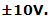The power fluctuation will be

WBJEE Physics Test - 2 - Question 4

The average power dissipation in a pure capacitor in AC circuit is

WBJEE Physics Test - 2 - Question 5

A thin spherical conducting shell of radius R has charge q. Another charge Q is placed at the centre of the shell. The electrostatic potential at a point P at a distance R/2 from the centre of the shell is

WBJEE Physics Test - 2 - Question 6

The length of an elastic string is 'a' meters when the longitudinal tension is 4N and 'b' meters when the longitudinal tension is 5N. The length of the string in meters when the longitudinal tension is 9N is

WBJEE Physics Test - 2 - Question 7

When two surfaces are coated with a lubricant, then they

WBJEE Physics Test - 2 - Question 8

The force of gravity is least at

WBJEE Physics Test - 2 - Question 9

The capacity of a condenser with two identical plates separated by a distance 'd' in air is 'C'. If the distance of separation is reduced to d 2 , the capacity is

WBJEE Physics Test - 2 - Question 10

A potential difference of 2V exists across a potentiometer wire of 2m length. When the potential difference across a 2Ω resistance of a second circuit is measured by this potentiometer wire, it amounts to 5mm balancing length. The current in the second circuit is

Detailed Solution for WBJEE Physics Test - 2 - Question 10

2.5 mA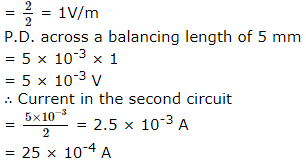WBJEE Physics Test - 2 - Question 11

An electron revolves in a circle of radius 0.4 Å with a speed of 106ms−1 in a hydrogen atom. The magnetic field produced at the centre of the orbit due to the motion of the electron in tesla is [μ0 = 4π x 10−7Hm−1 ; change on the electron = 1.6 x 10−19C]

WBJEE Physics Test - 2 - Question 12

If a diamagnetic solution is poured into a U-tube and one arm of this U-tube is placed between the poles of a strong magnet, with the meniscus in line with the field, then the level of solution will

WBJEE Physics Test - 2 - Question 13

Sixty four spherical rain drops of equal size are falling vertically through air with a terminal velocity 1.5 m-s⁻1. If these drops coalesce to form a big spherical drop, then terminal velocity of big drop is

WBJEE Physics Test - 2 - Question 14

A body of mass 0.4 kg is whirled in a vertical circle making 2 rev/sec. If the radius of the circle is 2 m, then tension in the string when the body is at the top of the circle, is

WBJEE Physics Test - 2 - Question 15

Which of the following is a vector quantity?

WBJEE Physics Test - 2 - Question 16

A radioactive element has a half life of 15 years. The fraction that will decay in 30 years is

WBJEE Physics Test - 2 - Question 17

Two masses m₁ and m₂ are suspended together by a massless spring of spring cosntant k. When the masses are in equilibrium, m₁ is removed without disturbing the system, then the angular velocity of oscillation of m₂ is

WBJEE Physics Test - 2 - Question 18

A body A going from south to North and body B is going from west to east. Then direction of relative velocity of A with respect to B is

WBJEE Physics Test - 2 - Question 19

Which one of the following statements is true for the speed (ν) and the acceleration (α) of a particle simple harmonic motion?

WBJEE Physics Test - 2 - Question 20

A convex lens of focal length 0.5 m and concave lens of focal length 1 m are combined .The power of resulting lens will be

WBJEE Physics Test - 2 - Question 21

From a uniform wire, two circular loops are made i) P of radius 'r' and ii)Q of radius nr. If the moment of inertia of Q about an axis passing through its centre and perpendicular to its plane is 8 times that of P about a similar axis, the value of 'n' is (diameter of the wire is very much smaller than r or nr)

WBJEE Physics Test - 2 - Question 22

The depletion of layer in the p-n junction region is caused by

WBJEE Physics Test - 2 - Question 23

If rotational kinetic energies of two bodies having moments of inertia of 9 kg-m2 and 1 kg-m2 are same, then ratio of their angular momenta is

Detailed Solution for WBJEE Physics Test - 2 - Question 23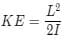since K.E. is same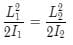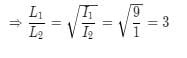WBJEE Physics Test - 2 - Question 24

Heat given to a body which rises its temperature by 1ºC is

WBJEE Physics Test - 2 - Question 25

The potential barrier, in the depletion of layer, is due to

WBJEE Physics Test - 2 - Question 26

A process in which volume remains constant is called

WBJEE Physics Test - 2 - Question 27

A unit vector perpendicular to each of the vectors (-6î+8k̂), (8î+6k̂) forming a right handed system is

WBJEE Physics Test - 2 - Question 28

Soap bubble looks coloured due to

WBJEE Physics Test - 2 - Question 29

The unit of electric flux is

WBJEE Physics Test - 2 - Question 30

A car moving with a speed of 40 km/h can be stopped by applying brakes after atleast 2m. If the same car is moving with a speed of 80 km/h, what is the minimum stopping distance ?

WBJEE Physics Test - 2 - Question 31

A 3 kg ball strikes a heavy rigid wall with a speed of 10 m/s at an angle of 60º .It gets reflected with the same speed and angle as shown .If the ball is in contact with the wall for 0.20 s, what is the average force exerted on the ball by the wall ?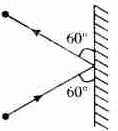WBJEE Physics Test - 2 - Question 32

A long, hollow conducting cylinder is kept coaxially inside another long, hollow conducting cylinder of larger radius.Both the cylinders are initially electrically neutral.

WBJEE Physics Test - 2 - Question 33

A copper cylindrical tube has inner radius a and outer radius b. The resistivity is ρ . The resistance of the cylinder between the two ends is

WBJEE Physics Test - 2 - Question 34

A car travels half the distance with constant velocity of 40 kmph and the remaining half with a constant velocity 60 kmph. The average velocity of the car in kmph is

*Multiple options can be correct
WBJEE Physics Test - 2 - Question 35

Two identical straight wires are stretched so as to produce 6 beats per second when vibrating simultaneouly. On changing the tension slightly in one of them, the beat frequency remains unchanged. Denoting by T1, T2 the higher and the lower initial tensions in the strings, then it could be said that while making the above changes in tension,

WBJEE Physics Test - 2 - Question 36

A charge q is placed at the centre of the joining two equal charges Q. The system of the three charges will be in equilibrium if q is equal to

*Multiple options can be correct
WBJEE Physics Test - 2 - Question 37

A square loop ABCD of edge 'a' moves to the right with a velocity v, parallel to AB. There is a uniform magnetic field of magnitude B, directed into the paper, in the region between PQ and RS only. I, II and III are three positions of the loop. Then,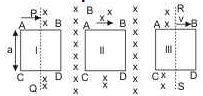WBJEE Physics Test - 2 - Question 38

A charge q is uniformly distributed along the periphery of a non-conducting uniform circular disc pivoted at its centre in a vertical plane and can freely rotate about a horizontal axis passing through its centre. A cylindrical region with its axis passing through the centre of the disc has a varying magnetic field B = kt as shown in the adjacent figure.
Here, k is a positive constant and t is time. A block of mass m is suspended from one end of a massless and inextensible string wrapped over the disc as shown and remains stationary, then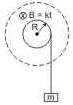Detailed Solution for WBJEE Physics Test - 2 - Question 38

*Multiple options can be correct
WBJEE Physics Test - 2 - Question 39

A conducting sphere of radius R, carrying charge Q, lies inside an uncharged conducting shell of radius 2R. If they are joined by a metal wire,

WBJEE Physics Test - 2 - Question 40

The area under force-displacement curve represents.

Detailed Solution for WBJEE Physics Test - 2 - Question 40
• The area under the force-displacement curve represents the work done.

• It is the distance (s) of the object displaced by the application of the force (f). In this case force (f) is presented on the y-axis and displacement (s) on the x-axis.

• The work done (w) is a scalar quantity.## WBJEE Sample Papers, Section Wise & Full Mock Tests

4 videos|10 docs|54 tests
 Use Code STAYHOME200 and get INR 200 additional OFF Use Coupon Code
Information about WBJEE Physics Test - 2 Page
In this test you can find the Exam questions for WBJEE Physics Test - 2 solved & explained in the simplest way possible. Besides giving Questions and answers for WBJEE Physics Test - 2, EduRev gives you an ample number of Online tests for practice

## WBJEE Sample Papers, Section Wise & Full Mock Tests

4 videos|10 docs|54 tests